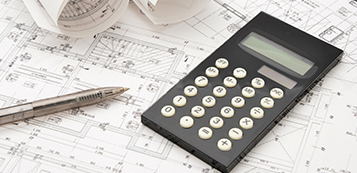# Ballpark Part EstimatorPart Volume

1. Enter the part volume in cubic inches. Most CAD packages will provide the part volume information.

EAU (Estimated Annual Usage)

1. If the EAU is less than 400 parts per year, the number you enter here will be the size of a single lot of the part. For example, if you enter 300, the estimated prices are based on a single lot of 300 parts.
2. If the EAU is equal to or greater than 400 parts per year, the EAU will be divided by four to give the estimated quarterly production lot. For example, if you enter 600, the estimated part prices are based on four lots of 150 each.
3. If you are looking at a single lot size greater than 400, just multiply that number by four to get the equivalent EAU. For example, if you want to estimate prices for a lot of 600 parts, multiply 600 by 4 and enter the EAU of 2400.